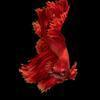## Welcome to the Treehouse Community

The Treehouse Community is a meeting place for developers, designers, and programmers of all backgrounds and skill levels to get support. Collaborate here on code errors or bugs that you need feedback on, or asking for an extra set of eyes on your latest project. Join thousands of Treehouse students and alumni in the community today. (Note: Only Treehouse students can comment or ask questions, but non-students are welcome to browse our conversations.)

### Looking to learn something new?

Treehouse offers a seven day free trial for new students. Get access to thousands of hours of content and a supportive community. Start your free trial today.

# how do i write a multiplication equation and is that an int, bool, etc?

i dont understand

types.swift
```// Enter your code below
let firstValue: Int = 5
let secondValue: Int = 3
let product: Int =
```Here is one solution:

```let firstValue = 5
let secondValue = 3
let product = firstValue * secondValue
let output = "The product of \(firstValue) times \(secondValue) is \(product)."
```First, you're doing great! You're almost there and Micah's code is what you're looking for to solve your problem. But seek understanding rather than copy/pasting the code, no matter how tempting it is to do otherwise.

Secondly, could you help us understand what it is that you aren't understanding? In the meantime...

You would want to use `Int` for integers/numbers, which is correct in the code you've provided. However, if you're multiplying numbers with decimals, you'd want to use `Double`, because you can't multiply variables or constants of two different types. In other words, the following code would give you an error because one is a double and the other is an int:

```let x: Int =  2
let y: Double = 3.15
let product = x * y
```

Of course there are ways around things, but don't worry about that yet. Focus on getting the basics down first.

You wouldn't want to use a `Bool` because that's for things that would evaluate to either `true`` or`false`` For example, say you could create some code that said a light switch is on:

```var lightSwitchIsOn: Bool = true
```

Now you have a variable that says the light switch is on. You'll learn about this more later, but then you could write more code that depending on whether the light swtich is on or not would perform a simple task. You could even write it to make `lightSwitchIsOn` evaluate to `false`, letting us know it's off.